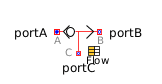Division - MapleSim Help

Complex Division

Output first input divided by second inputDescription The Complex Division (or Division) component computes the division of the two complex inputs ${u}_{1}$ and ${u}_{2}$. To use the complex conjugate of ${u}_{1}$ or ${u}_{2}$, set Use Conjugate Input 1 or Use Conjugate Input 2 to $\mathrm{true}$, respectively.Equations $y=\frac{{u}_{1}}{{u}_{2}}$Connections

 Name Description Modelica ID ${u}_{1}$ Complex input signal 1 u1 ${u}_{2}$ Complex input signal 2 u2 $y$ Complex output signal yParameters

 Name Default Units Description Modelica ID Use Conjugate Input 1 $\mathrm{false}$ If true, use the complex conjugate of input 1 useConjugateInput1 Use Conjugate Input 2 $\mathrm{false}$ If true, use the complex conjugate of input 2 useConjugateInput2Modelica Standard Library The component described in this topic is from the Modelica Standard Library. To view the original documentation, which includes author and copyright information, click here.